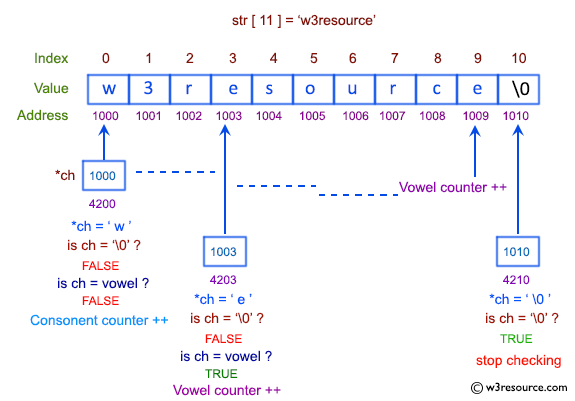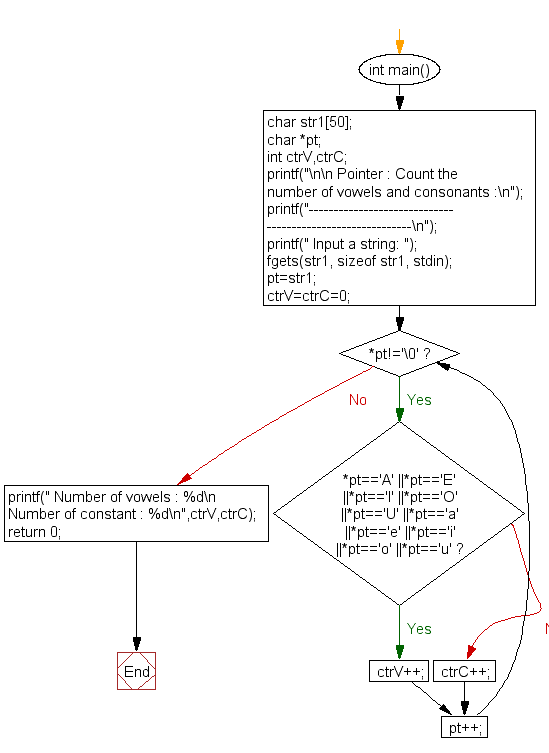﻿ C exercises: Count the number of vowels and consonants - w3resource# C Exercises: Count the number of vowels and consonants

## C Pointer : Exercise-13 with Solution

Write a program in C to count the number of vowels and consonants in a string using a pointer.

Pictorial Presentation:Sample Solution:

C Code:

``````#include <stdio.h>
int main()
{
char str1;
char *pt;
int  ctrV,ctrC;
printf("\n\n Pointer : Count the number of vowels and consonants :\n");
printf("----------------------------------------------------------\n");
printf(" Input a string: ");
fgets(str1, sizeof str1, stdin);

//assign address of str1 to pt
pt=str1;

ctrV=ctrC=0;
while(*pt!='\0')
{
if(*pt=='A' ||*pt=='E' ||*pt=='I' ||*pt=='O' ||*pt=='U' ||*pt=='a' ||*pt=='e' ||*pt=='i' ||*pt=='o' ||*pt=='u')
ctrV++;
else
ctrC++;
pt++; //pointer is increasing for searching the next character
}

printf(" Number of vowels : %d\n Number of consonants : %d\n",ctrV,ctrC-1);
return 0;
}
```
```

Sample Output:

```Pointer : Count the number of vowels and consonants :
----------------------------------------------------------
Input a string: string
Number of vowels : 1
Number of consonants : 5
```

Flowchart:C Programming Code Editor:

Have another way to solve this solution? Contribute your code (and comments) through Disqus.

What is the difficulty level of this exercise?

﻿

New Content: Composer: Dependency manager for PHP, R Programming